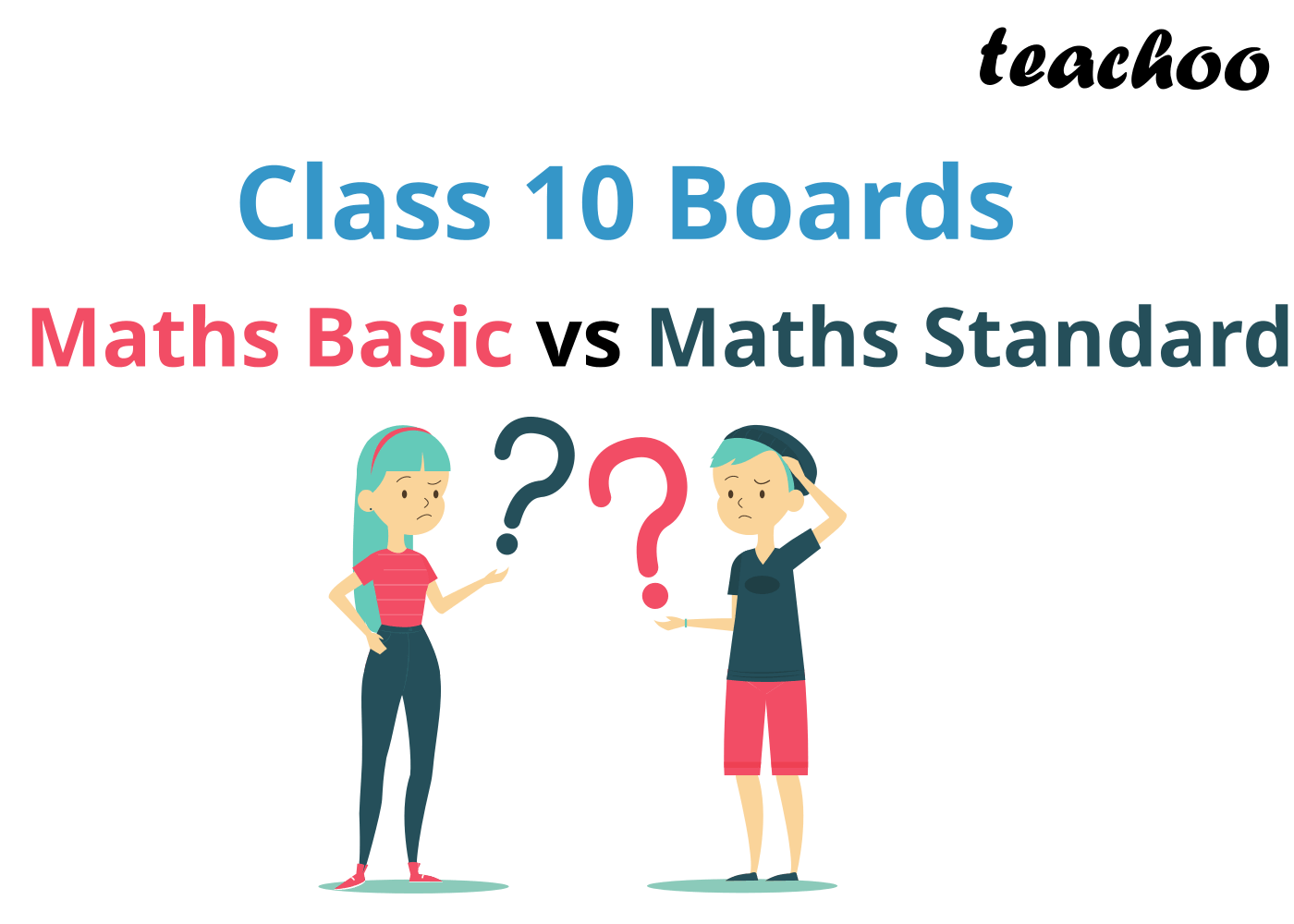# Maths Basic Vs Maths Standard - Class 10For March 2020 onwards, CBSE will conduct two Maths Exams for Class 10 Boards - Maths Basic and Maths Standard

Maths Basic is for students who do not want to pursue Maths in Class 11 and 12. While Maths Standard is compulsory for students who want to take up Maths in Class 11, 12 and College

The Maths Basic Exam is easier than the Maths Standard Exam.

We at Teachoo(टीचू) have listed all your queries about them in one place! Let's look at them one by one

## Are there two exams for Maths now?

No.

Students can choose if they want to give Maths Basic, or Maths Standard

The paper will be held in the same day.

## Is the Syllabus same in both Maths Basic and Maths Standard?

Yes.

The Syllabus of Maths Basic and Maths Standard exam is the same.

## What is difference in Maths Basic and Maths Standard?

The Maths Basic Exam is much easier than Maths Standard Exam.

## Can a student opt for both - Maths Basic and Maths Standard?

No.

Student can only solve 1 exam. She has to choose between - Maths Basic and Maths Standard

## Why do I choose Maths Standard over Maths Basic? The Basic one is easier

If you want to take up Maths in Class 11 and Class 12. Then take Maths Standard.

If you are sure you won’t study Maths in the future, take Maths Basic.

## What if I give the Basic Exam now, but want to appear for Standard Exam later?

If after giving the exam, you realise that you should have given the Standard Exam… then there’s an option.

You can give the Maths Standard Compartment Exam

## I am still not sure what exam to give, how can Teachoo help me?

Try solving the Sample Papers of both - Maths Basic and Maths Standard.

If the result in Maths Standard is really poor, you can do Maths Basic.

But, keep in mind.

If you take up Maths Basic, you cannot take Maths in Class 11 and 12.

Don't forget, you need to do NCERT Questions of Class 10, no matter which paper you take

## What if I fail in the exam?

There is an option to give Compartment Exams.

 Failed in Compartment Options Mathematics - Basic Mathematics - Basic Mathematics - Standard Mathematics - Standard or Mathematics - Basic

So, you can give Maths Basic Compartment Exam if you fail in Maths Standard

## What about the internal assessment of 20 marks?

Maths Exam is of total 100 marks, out of which 20 marks are internal assessment.

These 20 marks are for

• Internal Tests (10 Marks)
• Lab Report (5 Marks)
• Activities done in the lab (5 marks)

These 20 marks are the same for both Maths Basic and Maths Standard

## What about the Class 9 Exam?

The Class 9 exam is conducted by the schools and they will be the same for all students.

## To Summarise

Choose Maths Basic if you want to

• Take arts in Class 11 and 12
• Take Commerce without Maths in Class 11 and 12
• Take any non-maths course after Class 12 - like Design, Animation, Humanities

Choose Maths Standard if you want to

• Take Commerce with Maths in Class 11 and 12
• Take Science in Class 11 and 12
• Give IIT-JEE or Medical Exams (NEET)
• To do any Engineering course after Class 12
• To do CA, CS after Class 12
• Want to go to Medical Field after Class 12

No matter which you choose, you should do the Sample Papers and NCERT Solutions